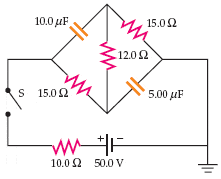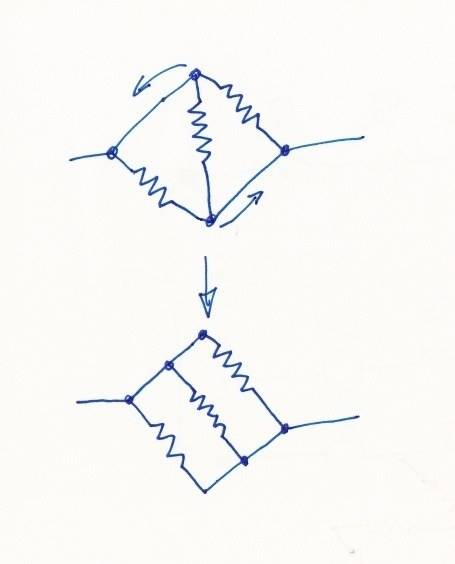# RC Circuit Question

## Homework Statement

Switch S, shown in the figure below, is closed after having been open for a long time.1) What is the initial value of the battery current just after switch S is closed?
2) What is the battery current a long time after switch S is closed?

V=IR C=Q/V

## The Attempt at a Solution

I really just need help redrawing this circuit so that I can see what is going on. I feel like if I can redraw it correctly, I will be able to understand how to do the problem. Can someone help me out with tips on how to redraw this specific circuit and similar ones in the future?[/B]

## Answers and Replies

Dr. Courtney
Education Advisor
Gold Member
2020 Award
Redrawing to recognize parallel and series elements can be of value.

But you also need a plan of attack beyond the three letter formulas.

Is this problem amenable to Kirchoff's laws, or do you need to solve differential equations once you get the picture simplified?

phinds
Science Advisor
Gold Member
I don't see how redrawing the circuit, in the sense of re-arranging the elements, is going to help. Just draw what the capacitors look like at t=0 and calculate the total resistance around the circuit. I suppose it might be a bit more clear if you re-arange the resistors to show clearly which are in series and which in parallel but that's pretty minor in this case.

Kirchoff's laws will help you solve this problem, but I don't see them as necessary for the first parts.

see, at t=0, I thought the current would flow through the upper capacitor, down the middle resistor, and back through the bottom capacitor. But instead it flows through everything and we have to use the fact that the resistors are all in parallel to find the total current. I don't understand why it's like that, and I think redrawing it would possibly help me understand.

CWatters
Science Advisor
Homework Helper
Gold Member
You don't need to redraw it much. Just replace the capacitors with the appropriate circuit element. What circuit element are you using?

So initially, the capacitors are just wires, right? Why is this thing in parallel with each component though?

CWatters
Science Advisor
Homework Helper
Gold Member
Have you actually redrawn it with the caps replaced by wires? If so just make the new wires shorter and move other bits around to suit and the answer should appear.

I could do a drawing but you should get it before I can do that.

I think redrawing helps to be able to discern which elements are in parallel.

When current can go through multiple parallel paths, it will divide up in such a way that the voltage across each of the parallel paths remains constant - as can be verified by Ohm's Law, KCL, and KVL.

CWatters
Science Advisor
Homework Helper
Gold Member
Looks like I got my drawing done first...•Luke Cohen
I think I got it now. The question I am struggling with is after a long time. What is the charge on the capacitors after a long time? I got that after a long time, the resistors are in series and we can calclate the total current based off that. From there, I used the current and resistor values to find the change in voltage in each branch of the circuit. Then I said that the change in voltage in the first 15O resistor is the same as the 10uF capacitor.But doing C=Q/V to find the charge game me an incorrect answer, so I don't know how to find the charge on the capacitors.

I think I got it now. The question I am struggling with is after a long time. What is the charge on the capacitors after a long time? I got that after a long time, the resistors are in series and we can calclate the total current based off that. From there, I used the current and resistor values to find the change in voltage in each branch of the circuit. Then I said that the change in voltage in the first 15O resistor is the same as the 10uF capacitor.But doing C=Q/V to find the charge game me an incorrect answer, so I don't know how to find the charge on the capacitors.

What are you using for V? Be careful. Think about what a capacitor looks like after a long time, and think about what the circuit looks like after a long time.

My change in V for the 10uF capacitor is equal to the 15O resistor multiplied by the total current found by using V=IR with all the resistors in series.

•EM_Guy
My change in V for the 10uF capacitor is equal to the 15O resistor multiplied by the total current found by using V=IR with all the resistors in series.

That looks right to me. So, then you should be able to get the voltage across each of your capacitors and the charge on each capacitor.

That's not correct according to my online physics program, though.

I figured it out. You have to use Kirchoff's loop rule. It's voltage doesn't just involve the resistor on the bottom, but it also involves the resistor in the middle.

CWatters
Science Advisor
Homework Helper
Gold Member
Deleted. I see you are trying to go beyond the original problem.